# Lecture 8 Product differentiation Product differentiation Standard models

• Slides: 9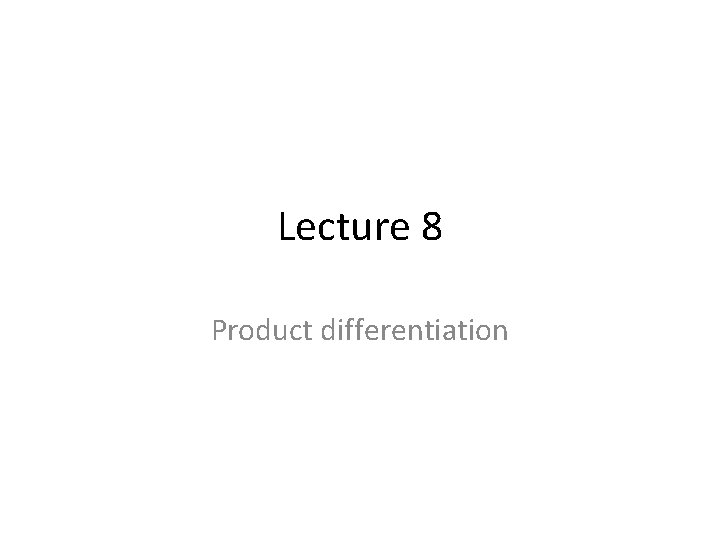Lecture 8 Product differentiation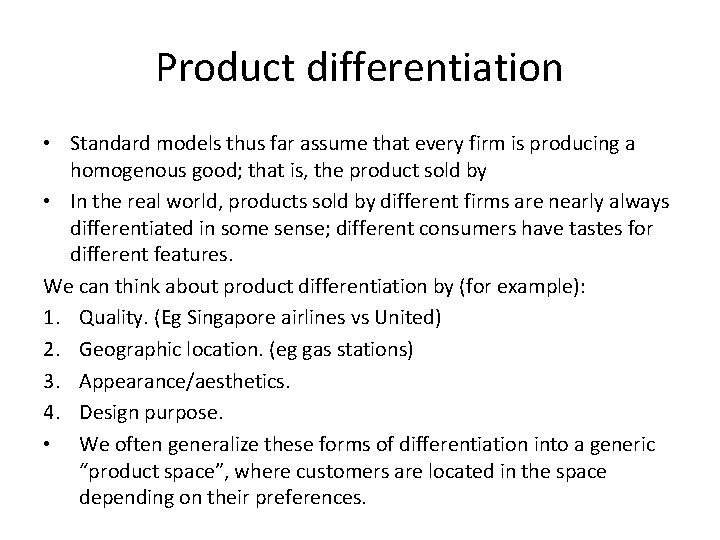Product differentiation • Standard models thus far assume that every firm is producing a homogenous good; that is, the product sold by • In the real world, products sold by different firms are nearly always differentiated in some sense; different consumers have tastes for different features. We can think about product differentiation by (for example): 1. Quality. (Eg Singapore airlines vs United) 2. Geographic location. (eg gas stations) 3. Appearance/aesthetics. 4. Design purpose. • We often generalize these forms of differentiation into a generic “product space”, where customers are located in the space depending on their preferences.Product quality • A firm might be able to produce a product of variable levels of quality. Consumers have preferences for higher quality products, but higher quality products are more expensive to produce. • Just as a firm has a tradeoff between increasing prices or increase quantity sold, a firm has a tradeoff between decreasing production costs and increasing quantity sold. • What level of quality should a firm choose to supply? It must be true in equilibrium that: 1. For a given choice of quality, the marginal revenue from the last unit sold must equal the marginal cost of making that unit at that quality. 2. For a given choice of quantity, the marginal revenue from increasing quality of each unit of output must equal the additional marginal cost of increasing the quality of that quantity of output.Product quality: example • Suppose that consumers have demand for a product given by: P = z(50 – Q) where z is the quality level of the product. • Suppose that the cost of quality is a sunk production cost, so the marginal production cost per unit is independent of the quality of the product. But the marginal cost of quality rises with the level of quality provided. To further simplify, assume that the marginal cost of producing the good is zero. • Specifically, assume: C(z, q) = F(z) = 5 z 2 • So the firm’s profit function is: π(Q, z) = P(Q, z)Q – F(z) = z(50 – Q)Q – 5 z 2.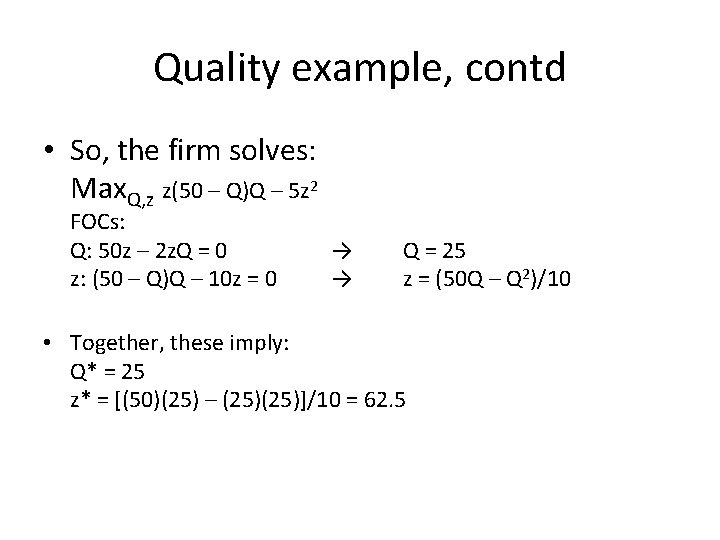Quality example, contd • So, the firm solves: Max. Q, z z(50 – Q)Q – 5 z 2 FOCs: Q: 50 z – 2 z. Q = 0 z: (50 – Q)Q – 10 z = 0 → → Q = 25 z = (50 Q – Q 2)/10 • Together, these imply: Q* = 25 z* = [(50)(25) – (25)]/10 = 62. 5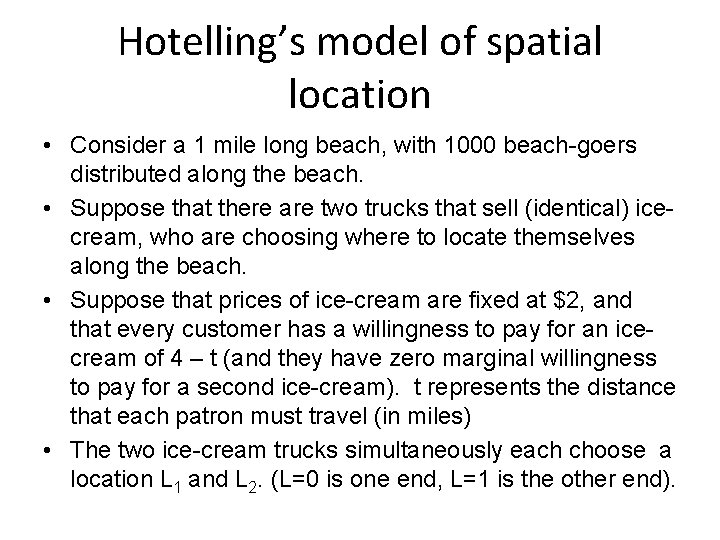Hotelling’s model of spatial location • Consider a 1 mile long beach, with 1000 beach-goers distributed along the beach. • Suppose that there are two trucks that sell (identical) icecream, who are choosing where to locate themselves along the beach. • Suppose that prices of ice-cream are fixed at \$2, and that every customer has a willingness to pay for an icecream of 4 – t (and they have zero marginal willingness to pay for a second ice-cream). t represents the distance that each patron must travel (in miles) • The two ice-cream trucks simultaneously each choose a location L 1 and L 2. (L=0 is one end, L=1 is the other end).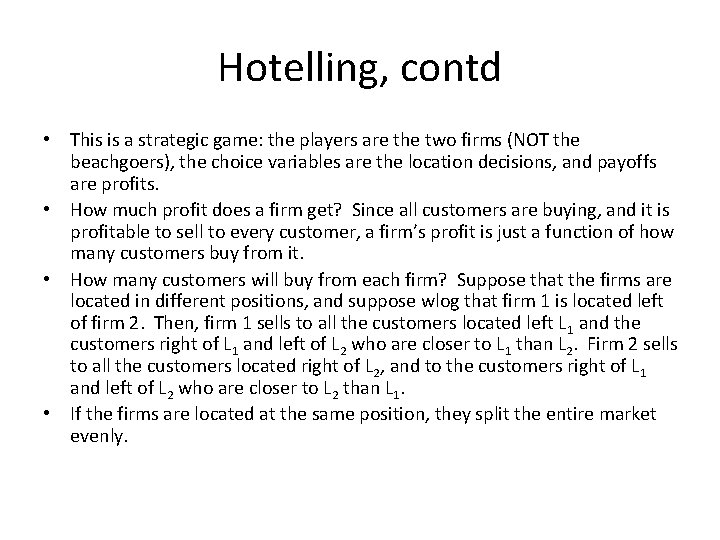Hotelling, contd • This is a strategic game: the players are the two firms (NOT the beachgoers), the choice variables are the location decisions, and payoffs are profits. • How much profit does a firm get? Since all customers are buying, and it is profitable to sell to every customer, a firm’s profit is just a function of how many customers buy from it. • How many customers will buy from each firm? Suppose that the firms are located in different positions, and suppose wlog that firm 1 is located left of firm 2. Then, firm 1 sells to all the customers located left L 1 and the customers right of L 1 and left of L 2 who are closer to L 1 than L 2. Firm 2 sells to all the customers located right of L 2, and to the customers right of L 1 and left of L 2 who are closer to L 2 than L 1. • If the firms are located at the same position, they split the entire market evenly.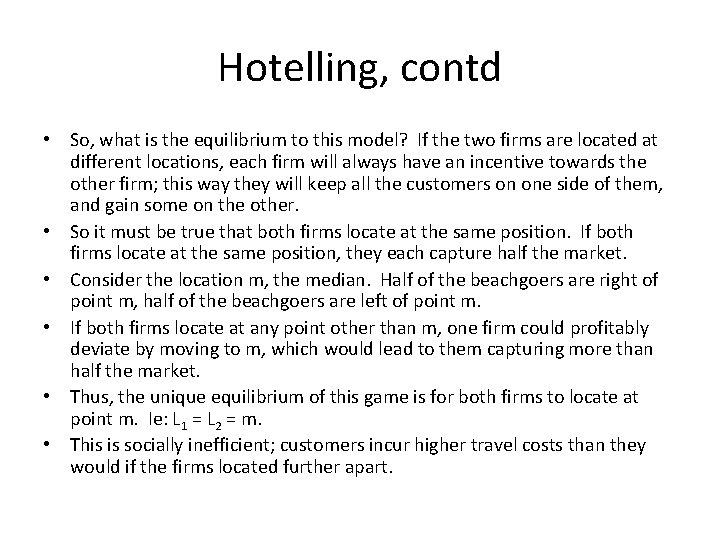Hotelling, contd • So, what is the equilibrium to this model? If the two firms are located at different locations, each firm will always have an incentive towards the other firm; this way they will keep all the customers on one side of them, and gain some on the other. • So it must be true that both firms locate at the same position. If both firms locate at the same position, they each capture half the market. • Consider the location m, the median. Half of the beachgoers are right of point m, half of the beachgoers are left of point m. • If both firms locate at any point other than m, one firm could profitably deviate by moving to m, which would lead to them capturing more than half the market. • Thus, the unique equilibrium of this game is for both firms to locate at point m. Ie: L 1 = L 2 = m. • This is socially inefficient; customers incur higher travel costs than they would if the firms located further apart.Hotelling, contd • Note that we did not need to assume anything about the distribution of beachgoers over the beach. If beachgoers are uniformly distributed, then m is the midpoint between the two ends, but this need not be the case. • This model gives a result where firms locate as close to each other as possible (actually at the same location). Other spatial models can have an equilibrium where firms locate as far away from each other as possible (Hotelling on a circle, rather than a line). • Other applications; Public choice theory (median voter theorem). Non-spatial product differentiation (imagine that the line represents preferences over some other product feature).